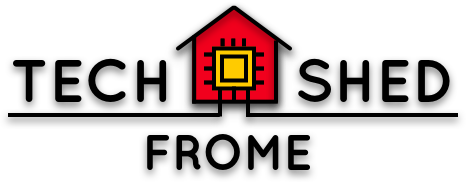Notes from our spotlight, written after the fact, so they may contain different material than we actually talked about.

• Functional language.
• Everything is a function! Even x = 3 is a function, of type Int.
• Implementation of the Lambda calculus, a formal model of computation.

# Why?

• Verifyability - we can formally check a program is correct
• Very strict typing - if it compiles, it probably works
• Determinism - given some input, a pure function always returns the same output. This makes it much easier to reason about programs (than when we have global variables, for example)
• Lazy Evaluation - computes just what is needed, and no more. Efficient, and allows us to write things that wouldn’t work in other languages (example).
• Clean and concise
• Can avoid imperative concepts such as loops and conditionals, which can be a source of confusion and bugs
• Learning Haskell makes everyone a better programmer!

# Coding Demo

Here is the code from the session, with extra comments.

``````-- A function that adds two integers together
add :: Int -> Int -> Int

-- add 2 to a number [ordinary version]
add2 x = x + 2

-- using currying: we can "half-apply" our original add function
-- add 2 :: Int -> Int

-- sum items in a list [pattern matching version]
sum1 :: [Int] -> Int
sum1 [] = 0 -- base case: empty list
sum1 (x:xs) = x + (sum1 xs) -- recursive case: x is the head of the list, xs is the tail (':' is called the "cons" operator)

-- how it works:
-- sum [1,2,3]
-- sum (1:[2,3]) = 1 + sum [2,3]
-- sum (2:3) = 2 + sum 
-- sum  = sum 3 + sum []
-- sum [] = 0
-- sum = 1 + 2 + 3 + 0

-- a second version using foldr (see http://www.zvon.org/other/haskell/Outputprelude/foldr_f.html)
sum2 :: [Int] -> Int
sum2 = foldr (+) 0

-- how it works
-- foldr f(x,y) base list
-- foldr (+) 0 xs
-- (+) :: Int -> Int -> Int

-- foldr f(x,y) base list
-- foldr f 0 []
-- 0
-- foldr f 0 [x1]
-- f(x1,0)
-- foldr f 0 [x1, x2]
-- f(x2,f(x1, 0))
-- let xs = [x1, x2, ..., xn]
-- foldr f 0 xs
-- f( ... f(x2, f(x1, 0))...)
``````
5 Likes

That looks great Hollie.